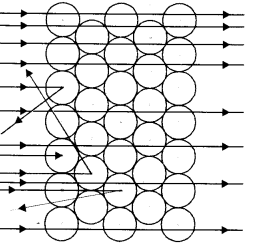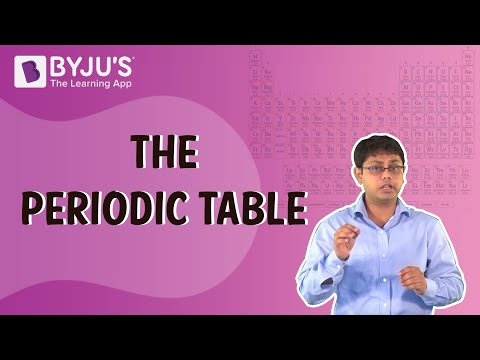Checkout JEE MAINS 2022 Question Paper Analysis : Checkout JEE MAINS 2022 Question Paper Analysis :

# Atomic Questions

The fundamental building block of chemistry is the atom. It is the smallest unit of matter that can be divided without releasing electrically charged particles. It is also the smallest unit of matter with the properties of a chemical element.

 Definition: Chemistry is based on the modern atomic theory, which states that atoms are the building blocks of all matter. Atoms are made up of protons, neutrons, and electrons. Each element has an atomic number that corresponds to the number of protons in its nucleus. The number of neutrons in an element’s isotopes varies. An atomic symbol is used to represent elements.

## Atomic Chemistry Questions with Solutions

Q1. Consider the species 72Zn, 75As and 74Ge. These species have:

(a) the same number of electrons.

(b) the same number of protons.

(c) the same number of neutrons.

(d) the same number of protons and neutrons.

Correct Answer. (c) the same number of neutrons.

Q2. Which model does not able to explain the stability of an atom?

(a) Bohr’s Model

(b) Rutherford’s Model

(c) Thomson’s Model

(d) None of the above

Q3. Experimentation with cathode-ray led to the discovery of-

(a) electrons

(b) protons

(c) neutrons

(d) nucleus

Q4. The principal quantum number describes-

(a) size of the orbital

(b) shape of the orbital

(c) spin of the orbital

(d) orientation of the orbital

Correct Answer – (a) size of the orbital

Explanation – Principal quantum number (n) determines the size of an orbital as- n = 1, 2, 3, 4, … and shells = K, L, M, N, …

Q5. Which of the following quantities can only be a whole number?

(b) Atomic number

(c) Maa number

(d) Equivalent weight

Correct Answer – (b) Atomic number

Q6. What are nucleons? What is the name given to those atoms which have a same number of nucleons in them?

Answer. A nucleon is a name given to two important subatomic particles: neutrons and protons.

ISOBARS is the name given to atoms that have the same number of nucleons.

Q7. How many unpaired electrons are there in a carbon atom in the ground state?

Answer. The electronic configuration of carbon is 1s2, 2s2, 2px1, 2py1. Therefore there are only 2 unpaired electrons in a carbon atom in the ground state.

Q8. What is the minimum number of quantum numbers required to specify an orbital? Name them.

Answer. There are three quantum numbers. These are as follows-

• Principal quantum no. (n)
• Azimuthal quantum No. (l)
• Azimuthal quantum No. (l)

Q9. Describe the important properties of cathode-rays. What is concluded about the nature of these rays?

Answer. The cathode rays have the following characteristics:

• Travel in straight lines perpendicular to the cathode’s surface.
• Material particles make up this substance.
• Have got the heating effect.
• It is made up of negatively charged particles.
• When they collide with hard metals such as copper, tungsten, or platinum, they emit X-rays.
• When they strike glass or certain other materials, such as zinc sulphide, they emit fluorescence.
• Permeate thin aluminium foils and other metals.
• Influence the photographic plates

Q10. What is meant by the dual nature of radiation?

Answer. The fact that light energy is carried in the form of energy packets (i.e., photons), as proposed by Planck’s theory, indicates that light has a particle character. At the same time, light has a wave-like character. These experimental findings led Einstein to propose that light has a dual nature, that it behaves both as a wave and as a particle.

Q11. An atom of an element has two electrons in the outermost M-shell. State its

(a) Electronic configuration

(b) Number of protons

(c) Atomic number

(d) Nature whether metal or non-metal

(e) Valency

(f) Name of the element.

(a) Since the atom has two electrons in the outermost M-shell, the K and L shells are already filled. As a result, the electronic configuration is 2, 8, 2. This means that the number of electrons of the atom is 12.

(b) The number of protons equals the number of electrons. Therefore, the number of protons will be 12.

(c) The atomic number equals the number of protons, which is 12.

(d) A metal. This is due to the fact that metallic elements have valence electrons less than 3.

(e) The element’s valency = the number of outermost electrons = 2.

(f) The element in question is magnesium (Mg).

Q12. Explain Bohr and Bury rules for the distribution of electrons into different shells.

• The maximum number of electrons that can be present in an atom’s energy shell is given by 2n2. ‘n’ denotes the number of energy shells or energy levels.
• According to the first rule, the outermost energy shell in an atom cannot have more than eight electrons, even if it has the capacity to accept more electrons.
• It is not necessary for a given shell to finish before another shell begins to form. In general, a new shell is formed when the outermost shell acquires eight electrons.

Q13. Define atomicity.

Answer. The number of atoms in an element’s molecule is referred to as its atomicity.

It is classified as follows:

• Monoatomic molecules: Xenon is an example of a monoatomic molecule (Xe)
• Diatomic molecule: a molecule with two atoms, such as oxygen (O2).
• Triatomic molecule: a molecule with three atoms, such as ozone (O3).
• Tetratomic molecule: a molecule with four atoms, such as phosphorus (P4).
• Polyatomic molecule: a molecule with more than four atoms, such as sulphur (S8).

Q14. Define the terms

(a) isotope

(b) isobar giving one example in each case.

(c) Name the element whose isotope is used in

(i) nuclear reactor,

(ii) treatment of cancer.

(a) The majority of the elements can be found in nature. Some atoms of a given element may have different mass numbers. Their atomic numbers, however, are the same. These are known as isotopes. Isotopes are thus defined as different atoms of the same element with the same atomic number but different mass numbers.

(b) Isobars are atoms from different elements that have the same mass number but different atomic number.

(c)

(i) The U-235 isotope is used as a fuel in nuclear reactors.

(ii) Co-60 isotope is used in cancer treatment.

Q15. Write any five features of Dalton’s atomic theory.

Answer. The five features of Dalton’s atomic theory are as follows-

• Every substance is made up of extremely small particles known as atoms.
• Atoms are the ultimate matter particles and cannot be subdivided into smaller particles.
• Atoms cannot be created or destroyed during a physical or chemical change.
• All atoms of a given element are identical in every way. This means they have the same mass, size, and chemical properties.
• Different elements’ atoms have different masses, sizes, and chemical properties.

## Practise Questions on Atomic

Q1. Which of the following quantum numbers determines an electron’s spin direction?

(a) n

(b) l

(c) m

(d) s

Q2. Name the element which is used as the reference for atomic mass.

(a) Carbon

(b) Oxygen

(c) Helium

(d) Chlorine

Q3. Using s, p, d notations, describe the orbital with the following quantum numbers.

(a) n=1, l=0;

(b) n = 3; l=1;

(c) n = 4; l =2;

(d) n=4; l=3.

Q4. An atom has 2 K, 8 L, and 5 M electrons. Write down the atom’s electronic configuration. What is the number of unpaired electrons in the atom?

Q5. a) Which popular experiment is shown in the figure?(b) List three observations of this experiment.

(c) State conclusions drawn from each observation of this experiment.

(d) State the main features of the above experiment.

Click the PDF to check the answers for Practice Questions.

## Recommended Videos

#### The Periodic Table#### Size of an Atom#### The Gold Foil Experiment